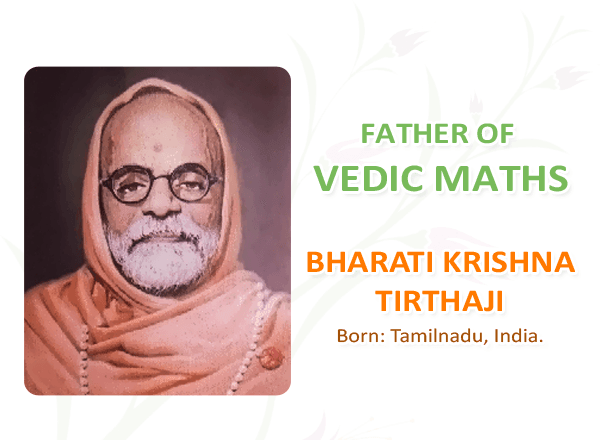Search

# What is Vedic Mathematics?• Vedic Mathematics is a collection of Techniques/Sutras to solve mathematical arithmetics in easy and faster way. It consists of 16 Sutras (Formulae) and 13 sub-sutras (Sub Formulae) which can be used for problems involved in arithmetic, algebra, geometry, calculus, conics.

• Vedic Mathematics is a system of mathematics which was discovered by Indian mathematician Jagadguru Shri Bharathi Krishna Tirthaji in the period between A.D. 1911 and 1918 and published his findings in a Vedic Mathematics Book by Tirthaji Maharaj

• Veda is a Sanskrit word which means ‘Knowledge’.

• Using regular mathematical steps, solving problems sometimes are complex and time consuming. But using Vedic Mathematic’s General Techniques (applicable to all sets of given data) and Specific Techniques (applicable to specific sets of given data), numerical calculations can be done very fast.

## History Of Vedic MathematicsShri Bharathi Krishna Tirthaji Maharaj was born in March 1884 in the Puri village of Orissa state. He was very good in subjects like mathematics, science, humanities and was excellent in Sanskrit language. His interests were also in spiritualism and mediation. In fact when he was practicing meditation in the forest near Sringeri, he rediscovered the Vedic sutras. He claims that these sutras/techniques he learnt from the Vedas especially ‘Rig-Veda’ directly or indirectly and he intuitively rediscovered them when he was practicing meditation for 8 years.

Later he wrote the sutras on the manuscripts but were lost. Finally in year 1957, he wrote introductory volume of 16 sutras which is called as Vedic Mathematics and planned to write other sutras later. But soon he developed cataract in both of his eyes and passed away in year 1960.

## How Vedic Mathematics is Beneficial and What are the Advantages of Vedic MathematicsVedic Mathematics can definitely solve mathematical numerical calculations in faster way. Some Vedic Math Scholars mentioned that Using Vedic Maths tricks you can do calculations 10-15 times faster than our usual methods. I agree this to some extent because some methods in Vedic Mathematics are really very fast. But some of this methods are dependent on the specific numbers which are to be calculated. They are called specific methods.